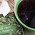## Friday, July 1, 2016

### Werewolf's Tale and a Druid's Sword by Lexi Ander - Cover Reveal with Giveaway=•=•=•=•=•=•=•=•=•=•=•=•=•=•=•=•=•=•=•=•=•=•=•=•=•=•=•=•=

Lexi Ander reveals the beautiful cover art of her next book titled WEREWOLF’S TALE AND A DRUID’S SWORD coming out from Less Than Three Press.

It releases on August 31, 2016.

=•=•=•=•=•=•=•=•=•=•=•=•=•=•=•=•=•=•=•=•=•=•=

BLURB

After he's kicked out for being gay, still reeling from being abandoned by the man he loves, Jude goes to live with his brother Beck, who thankfully is more than happy to take him in. But Jude has other secrets he's yet to share, for fear that even Beck will reject him, leaving him well and truly alone.
Then he draws the attention of werewolves, and discovers that everything he thought he knew about Levi, the man who abandoned him, is a lie. But one lie leads to another, ending in a vast conspiracy that threatens to destroy everything Jude loves—and that doesn't even begin to include the challenges that Beck is facing.

=•=•=•=•=•=•=•=•=•=•=•=•=•=•=•=•=•=•=•=•=•=•=•=•=•=•=•=•=

PRESENTING

Cover Art by Aisha Akeju=•=•=•=•=•=•=•=•=•=•=•=•=•=•=•=•=•=•=•=•=•=•=

TEASER

Coming from Boston, he thought the fall evenings in San Diego were nice. He couldn't help but laugh a little at the Californians who bundled up when temperatures dropped to sixty degrees. A strange noise drew his attention to the corner of the condo. Thick shadows draped the far side of the building in inky darkness. The sound came again, snuffling then a whimper. Jude wondered if someone's pet dog was loose. He considered what he could do to help the pooch when the whimper turned into a hair-raising growl. Jude's heart skipped a beat at the low sound. Then again, there were more things that went bump in the night than stray pets. Nope. Being mauled on the front lawn at eleven in the evening didn't factor into Jude's night activities.

He spun on his heel and retreated into the house. By Friday-night standards, the hour was early. When he pushed the door open, Beck was waiting there and crushed him into a bear hug.

=•=•=•=•=•=•=•=•=•=•=•=•=•=•=•=•=•=•=•=•=•=•=Lexi has always been an avid reader, and at a young age started reading (secretly) her mother’s romances (the ones she was told not to touch). She was the only teenager she knew of who would be grounded from reading. Later, with a pencil and a note book, she wrote her own stories and shared them with friends because she loved to see their reactions. A Texas transplant, Lexi now kicks her boots up in the Midwest with her Yankee husband and her eighty-pound puppies named after vacuum cleaners.

||  Website  ||  Blog  ||  Facebook  ||  Twitter  ||  Email  ||  Amazon Author Page  ||

=•=•=•=•=•=•=•=•=•=•=•=•=•=•=•=•=•=•=•=•=•=•=

RAFFLECOPTER GIVEAWAY

Winner’s Prize: \$15 Amazon Gift Card
Runner Up Prize: \$10 Amazon Gift Card
2nd Runner Up Prize: \$5 Amazon Gift Card

#### 1 comment:

1.Love the cover, it's on the wave with the title. And that blurb sure did intrigued me. Can't wait for August!